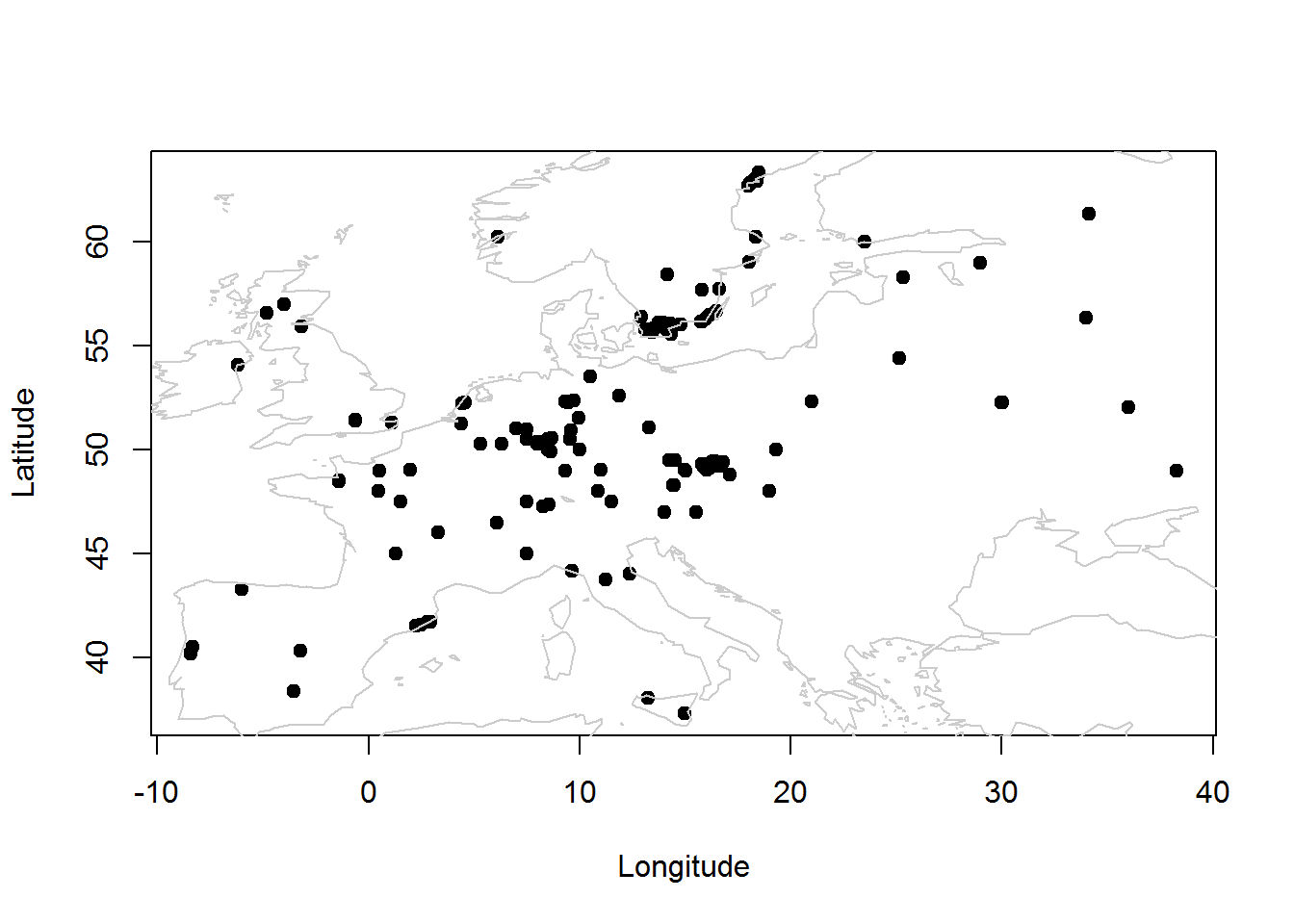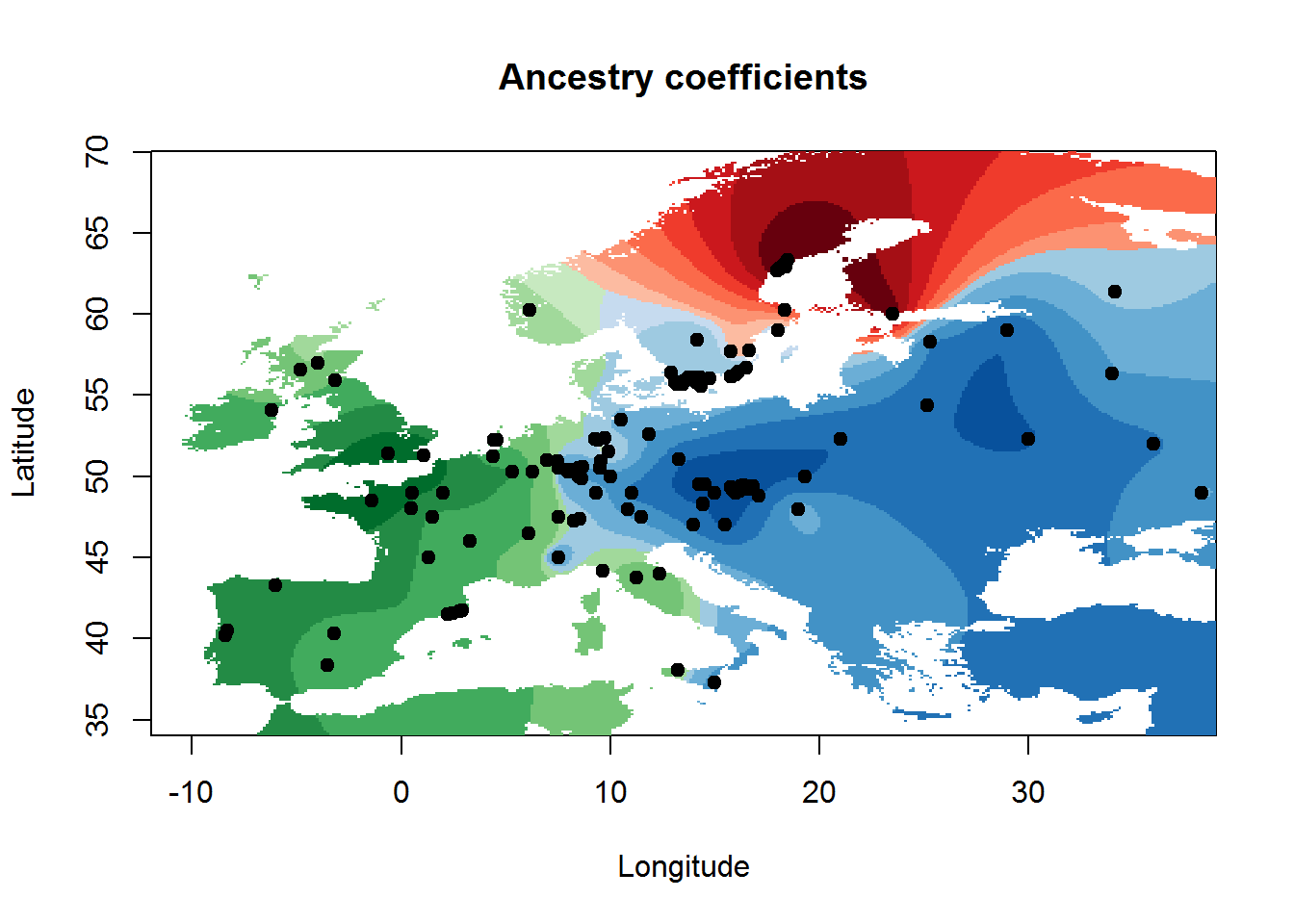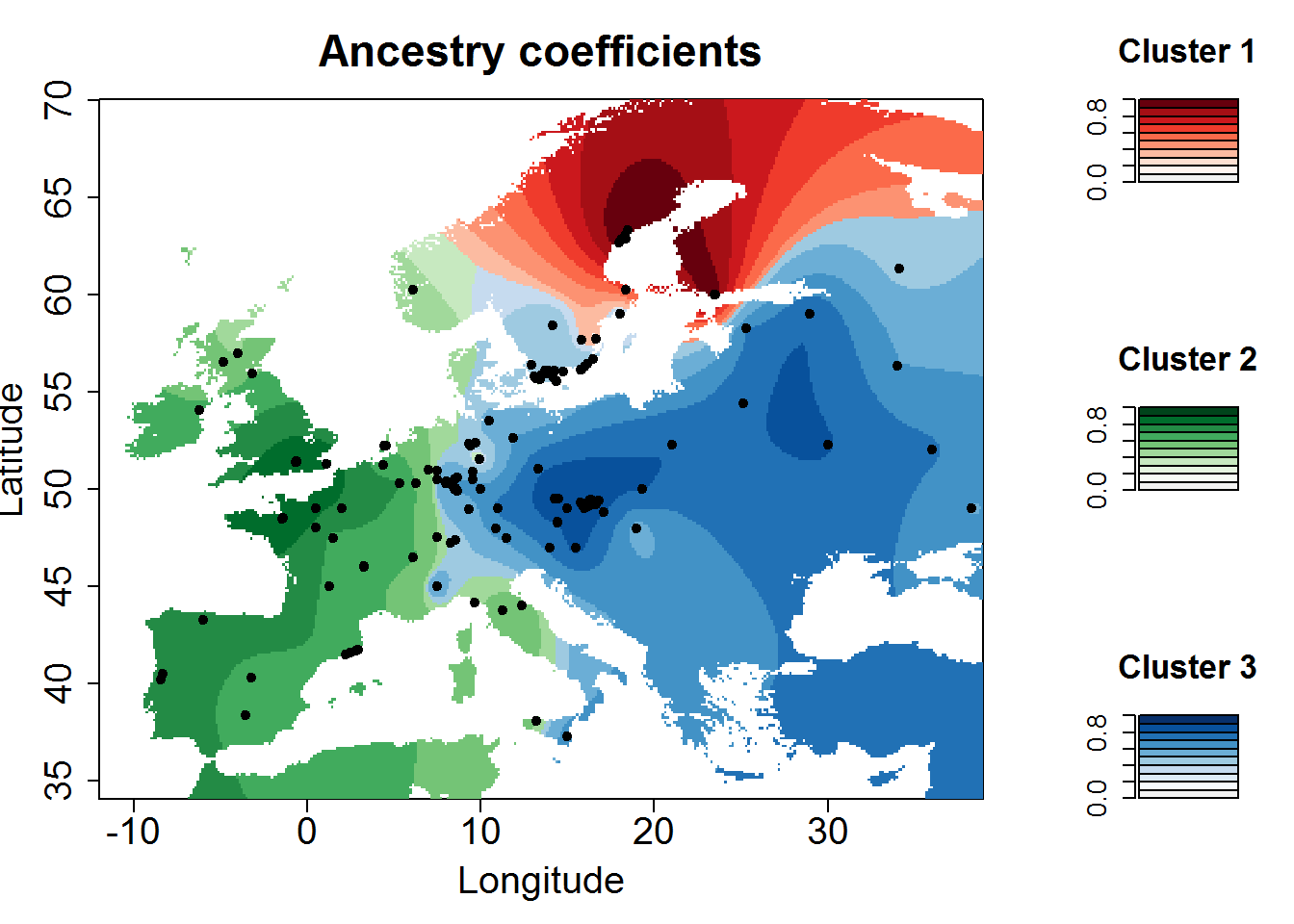This page illustrates how to display a $$Q$$ matrix formed by $$K$$ vectors of ancestry coefficients within a geographic map using an ascii raster file of the study area. The script works fine for $$K$$ less than 6, and it can be modified to work with values greater than 6. Then, users will have to specify a new color palette.

The following example displays an interpolated map for the ancestry coefficients of 170 European lines of the plant species Arabidopsis thaliana. The genetic data were published in (Atwell et al., Science, 2010). The $$Q$$ matrix was computed using TESS3 using $$K = 3$$ clusters. We also applied the snmf function from the R package LEA to the genetic data, and it led to very similar results.

Buiding the geographic map requires 3 files:

1. A file for the geographic coordinates of each of the $$n = 170$$ individuals.The files records the longitude and latitude of each individual (Lon, Lat) in a matrix format. In our example, the geographic coordinate file has a .coord extension, but any other extension is allowed.

2. A file for the ancestry coefficients of each individual ($$Q$$-matrix). Here, the $$Q$$-matrix can be found in the “Athaliana_k3.Q” file. Each column represents an ancestral population, and each line corresponds to the $$K = 3$$ individual coefficients.

3. An ascii raster file for the study area (low resolution map), “lowResEurope.asc”.

The 3 files can be downloaded from the zipped archive available here: MapDisplay.zip. For application of the method to your own geographic data, raster files are available for major geographic regions of the world here RasterMaps.zip.

The method presented below also works well for the output files of several programs including TESS, TESS3, POPS, sNMF, STRUCTURE and CLUMPP, and any other program that computes $$Q$$-matrices.

The example is based on an R script written by Flora Jay for POPS output files (Jay et al. Mol. Ecol. 2012). This script is available from the POPS web page http://membres-timc.imag.fr/Olivier.Francois/pops.html and it can be downloaded here: POPSutilities.R

After having uncompressed the zip file, our R session starts with sourcing Flora’s script.

source("MapDisplay/POPSutilities.R")
## Warning in helpPops(): Available functions:
##
##  HELP:
##    * helpPops()
##
##
##  SHOW EXAMPLE:
##    * Open the R script scriptExample.r
##
##
##  CORRELATION UTILITIES:
##  Compute correlation between matrix of membership/admixture coefficients (from matrix or from POPS outputs)
##    * correlation(matrix1,matrix2,plot=TRUE,colors=defaultPalette)
##    * correlationFromPops(file1,file2,nind,nskip1=2,nskip2=2,plot=TRUE,colors=defaultPalette)
##
##
##  BARPLOT UTILITIES:
##  Display barplot of membership/admixture coefficients (from matrix or from POPS output)
##  * barplotCoeff(matrix,colors=defaultPalette,...)
##  * barplotFromPops(file1,nind,nskip1=2,colors=defaultPalette,...)
##
##
##  MAPS UTILITIES:
##  Display maps of membership/admixture coefficients (from matrix or from POPS output)
##  Create grid on which coefficients will be displayed
##    * createGrid(min_long,max_long,min_lat,max_lat,npixels_long,npixels_lat)
##    * createGridFromAsciiRaster(file)
##    * getConstraintsFromAsciiRaster(file,cell_value_min=NULL,cell_value_max=NULL)
##  Legend for maps
##    * displayLegend(K=NULL,colorGradientsList=lColorGradients)

Note that, depending on which packages are installed on the system, it might be necessary to repeat this operation twice.

The programs TESS, POPS, STRUCTURE and CLUMPP share the same format for Q-matrices. The programs TESS3 and sNMF share the same format, but their format is different from the other programs.

The TESS3 and sNMF format consist of a matrix with $$n$$ rows (each row corresponds to an individual) and $$K$$ columns (each column corresponds to an ancestral population). Here we read a $$Q$$-matrix with $$K=3$$ columns in the simplest format (TESS3,sNMF). We load a $$Q$$-matrix computed by TESS3.

Qmatrix = read.table("MapDisplay/Athaliana_k3.Q") 

An example of the TESS 2.3 format can be found here http://membres-timc.imag.fr/Olivier.Francois/outfile.txt. We see that it is easy to obtain the $$Q$$-matrix from this output format after removing the first and the last columns. The STRUCTURE format is similar to TESS 2.3. So the above comments also applies to STRUCTURE output files.

The geographic coordinates can be read as follows

coord = read.table("MapDisplay/Athaliana.coord")
plot(coord, pch = 19,  xlab="Longitude", ylab = "Latitude")
map(add = T, interior = F, col = "grey80")and our ascii raster file for the European continent is

asc.raster = "MapDisplay/lowResEurope.asc" 

In this raster file, “XLLCENTER”, “YLLCENTER” can be replaced by “XLLCORNER”, “YLLCORNER” without changing the results. Check that “NODATA_VALUE” is set to some value (e.g., “NODATA_VALUE -9999”“).

The following R commands define a grid on which the script will interpolate the ancestry coefficients

grid=createGridFromAsciiRaster(asc.raster)

For removing values that fall below the sea level, then use cell_value_min=0 when specifying the constraints on the grid.

constraints=getConstraintsFromAsciiRaster(asc.raster,cell_value_min=0)

Then a basic map is obtained using the maps function of the R script.

maps(matrix = Qmatrix, coord, grid, constraints, method = "max", main = "Ancestry coefficients", xlab = "Longitude", ylab = "Latitude")Now, suppose that we want to include keys for grading the colored clusters displayed in the map. This can be done as follows.

show.key = function(cluster=1,colorGradientsList=lColorGradients){
barplot(matrix(rep(1/10,10)),col=colorGradientsList[[cluster]][(ncolors-9):ncolors],main=paste("Cluster",cluster))}
layout(matrix(c(rep(1,6),2,3,4), 3, 3, byrow = FALSE), widths=c(3,1), respect = F)
for(k in 1:3){show.key(k)}